Analytical & Nuclear Chemistry

# Analytical & Nuclear Chemistry

Test Description

## 20 Questions MCQ Test GATE Chemistry Mock Test Series | Analytical & Nuclear Chemistry

Analytical & Nuclear Chemistry for Chemistry 2022 is part of GATE Chemistry Mock Test Series preparation. The Analytical & Nuclear Chemistry questions and answers have been prepared according to the Chemistry exam syllabus.The Analytical & Nuclear Chemistry MCQs are made for Chemistry 2022 Exam. Find important definitions, questions, notes, meanings, examples, exercises, MCQs and online tests for Analytical & Nuclear Chemistry below.
Solutions of Analytical & Nuclear Chemistry questions in English are available as part of our GATE Chemistry Mock Test Series for Chemistry & Analytical & Nuclear Chemistry solutions in Hindi for GATE Chemistry Mock Test Series course. Download more important topics, notes, lectures and mock test series for Chemistry Exam by signing up for free. Attempt Analytical & Nuclear Chemistry | 20 questions in 60 minutes | Mock test for Chemistry preparation | Free important questions MCQ to study GATE Chemistry Mock Test Series for Chemistry Exam | Download free PDF with solutions
 1 Crore+ students have signed up on EduRev. Have you?
*Answer can only contain numeric values
Analytical & Nuclear Chemistry - Question 1

### The distribution coefficient of an organic compound A for benzene and water is 10. The amount of A extracted if 1.0 gm of it dissolved in 100 mL of water is equilibrated in a separatory funnel with 100 mL of benzene is ______ gm. (Round off to two one decimal place)

Detailed Solution for Analytical & Nuclear Chemistry - Question 1

Suppose amount of A extracted with 100 mL of benzene = xg. The amount of A left in 100 mL water = 1–xg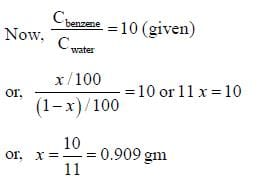The amount of A extracted with 100 mL of benzene is 0.909 gm.

*Answer can only contain numeric values
Analytical & Nuclear Chemistry - Question 2

### Substances X and Y were found to have a retention time of 18.30 and 29.92 minutes respectively on a 20 cm long column. The widths (at the base) for A and B were 1.50 and 1.60 minutes respectively. The column resolution is ____. (Round off to two decimal places).

Detailed Solution for Analytical & Nuclear Chemistry - Question 2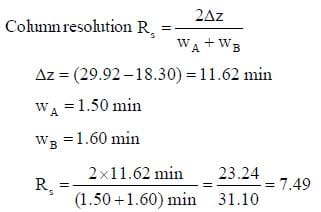Analytical & Nuclear Chemistry - Question 3

### In differential thermal analysis (DTA) the correct representation of ΔT (temperature) difference between sample and thermally inert reference are continuously recorded as a function of temperature or time.

Detailed Solution for Analytical & Nuclear Chemistry - Question 3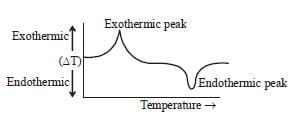Analytical & Nuclear Chemistry - Question 4

The correct form of half wave potential in a typical polarogram.

Detailed Solution for Analytical & Nuclear Chemistry - Question 4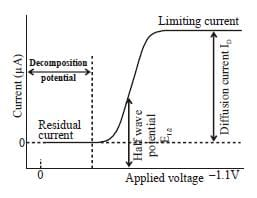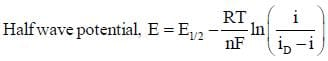Analytical & Nuclear Chemistry - Question 5

The major limitations of quinhydrone electrode are

Detailed Solution for Analytical & Nuclear Chemistry - Question 5

All are correct. In alkaline solution (above pH 9) the weakly acidic  is neutralised and is subject to oxidation by air and dissolved oxygen.

Analytical & Nuclear Chemistry - Question 6

Which of the following metals can be used as indicator electrodes in potentiometric methods

Detailed Solution for Analytical & Nuclear Chemistry - Question 6

Metals that undergo reversible oxidation

Analytical & Nuclear Chemistry - Question 7

Which of the following statements are correct for a successful mass spectroscopic analysis
1. Each component must exhibit atleast one peak that differs makedly from the others
2. The contribution of each component to a peak must be linearly additive
3. The sensitivity must be reproducible to 1% relative
4. Suitable standards must be available for calibration

Detailed Solution for Analytical & Nuclear Chemistry - Question 7

All are correct, sensitivity is defined as ion current per unit partial pressure.

Analytical & Nuclear Chemistry - Question 8

U235 nucleus splits into two new nuclei whose mass numbers are in the ratio of 2 : 1. The ratio of the radii of the new nuclei is

Detailed Solution for Analytical & Nuclear Chemistry - Question 8

The ratio of radii of two nuclei: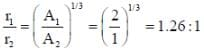Analytical & Nuclear Chemistry - Question 9

Half life of Na24 radionuclide is 15h the time in which the activity of a sample of  Na24 radionuclide will decrease by 87.5% is

Detailed Solution for Analytical & Nuclear Chemistry - Question 9

Let the initial activity of sample is 100 u. Then from question the final activity should be 100 – 87.5 = 12.5 u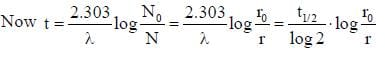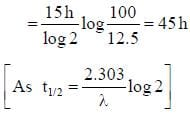Analytical & Nuclear Chemistry - Question 10

The number of α and β particles are emitted out in the transition 92U238 to 82Pb206 are

Detailed Solution for Analytical & Nuclear Chemistry - Question 10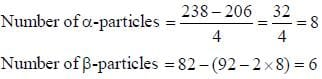*Answer can only contain numeric values
Analytical & Nuclear Chemistry - Question 11

Y90 has a half-life of 64h and Sr90 28 year. Sr90 by β-emission the amount of Y90 in equilibrium with 1 gm of Sr90 is ___  x 10-4 gm. (Round off to two decimal places)

Detailed Solution for Analytical & Nuclear Chemistry - Question 11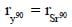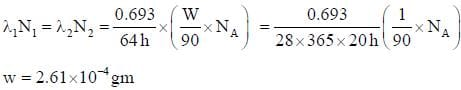Analytical & Nuclear Chemistry - Question 12

A cell voltage measured using an SCE reference electrode is –0.774 V. (The indicates electrode is more negative half cell). The cell voltage with a Ag/AgCl reference electrode (1 MKCl : E = 0.228 V) or with NHE are

Detailed Solution for Analytical & Nuclear Chemistry - Question 12

The potential of Ag/AgCl electrode is more negative than that of the SCE by 0.242 – 0.228 = 0.014 V Hence the cell voltage using the former electrode is less negative by this amount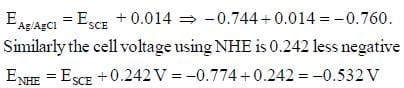Analytical & Nuclear Chemistry - Question 13

Which of the following forms of analytical chemistry seeks to obtain the condition of full polarization

Detailed Solution for Analytical & Nuclear Chemistry - Question 13

Voltametry
Correct options is (b).

Analytical & Nuclear Chemistry - Question 14

Compared to AAS, AES has the advantage of providing

Detailed Solution for Analytical & Nuclear Chemistry - Question 14

Because of the excitation source is general, all atoms are atomized, excited, and product atomic emission.

Analytical & Nuclear Chemistry - Question 15

Which statement is FALSE about light source

Detailed Solution for Analytical & Nuclear Chemistry - Question 15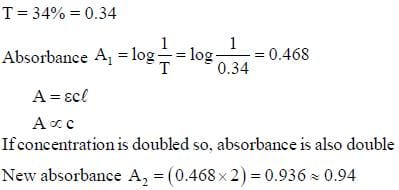Analytical & Nuclear Chemistry - Question 16

1.0 gram of a sample of  H2O2 solution containing y% H2O2 by weight requires y mL of KMnO4 solution for complete titration under acidic condition. The molarity of KMnOsolution is

Detailed Solution for Analytical & Nuclear Chemistry - Question 16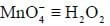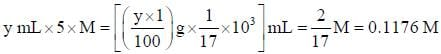Analytical & Nuclear Chemistry - Question 17

Which type of chromatography process requires that when materials that cannot be readily dissolved in a solvent for chromatography need to be heated or pyrolyzed to a high temperature is

Detailed Solution for Analytical & Nuclear Chemistry - Question 17

Gas chromatography
Correct option is (b).

Analytical & Nuclear Chemistry - Question 18

Differential scanning calorimetry (DSC) is a technique useful in determining

Detailed Solution for Analytical & Nuclear Chemistry - Question 18

glass transition temperatures

Analytical & Nuclear Chemistry - Question 19

The best procedure to improve resolution between two chromatographic peak is

Detailed Solution for Analytical & Nuclear Chemistry - Question 19

decreasing column length, decreasing band-width
Correct option is (d).

Analytical & Nuclear Chemistry - Question 20

In paper chromatographic separation of silver, lead and Hg the solvent front was 18 cm while front due these elements were respectively 16, 12 and 6 cm. What is the Rf value of all metals.

Detailed Solution for Analytical & Nuclear Chemistry - Question 20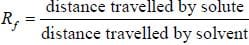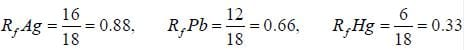## GATE Chemistry Mock Test Series

1 docs|34 tests
 Use Code STAYHOME200 and get INR 200 additional OFF Use Coupon Code
Information about Analytical & Nuclear Chemistry Page
In this test you can find the Exam questions for Analytical & Nuclear Chemistry solved & explained in the simplest way possible. Besides giving Questions and answers for Analytical & Nuclear Chemistry, EduRev gives you an ample number of Online tests for practice

1 docs|34 tests